# A barn manager has designed a circular enclosure with a circumference of 75.4 feet. She would like to order sand to fill the enclosure. If one unit of sand covers 27 square feet. How many units of sand will she need to order? Please round your answer up to the nearest whole unit.

17 units

16.75 units

16 units

20 units

452 sq ft

17 units

Explanation:

First, you will need to work backward from the circumference to find the radius of the circular enclosure.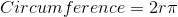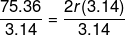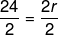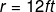Now we know what the radius is, we can calculate the surface area of the floor of the enclosure.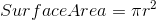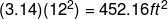Finally, we need to find the number of units of sand needed to cover the floor of the enclosure.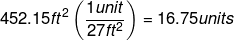### Example Question #2 : Diameter, Radius, And Circumference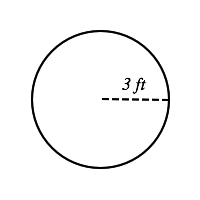# Find the diameter, circumference and area of the circle above.

Diameter= 6 ft

Circumference= 19 ft

Area= 30 ft2

Diameter= 6 ft

Circumference=18.84 ft

Area= 28.27 ft2

Diameter= 3ft

Circumference=37.68 ft

Area= 28.7 ft2

Diameter= 6ft

Circumference=37.68 ft

Area= 28.27 ft2

Diameter= 9ft

Circumference=37.68 ft

Area= 28 ft2

Diameter= 6 ft

Circumference=18.84 ft

Area= 28.27 ft2

Explanation:

To find the diameter, you must know that radius is half the diameter (or the diameter is 2 times the radius.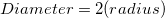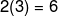### The diameter is 6ft.

To find the circumference, you must multiply the diameter (6ft) by pi.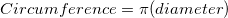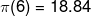### The circumference is 18.84 ft.

To find the surface area, you must aquare the radius (3ft) and multiply by pi.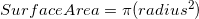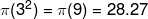### Example Question #3 : Diameter, Radius, And Circumference

A circle has a diameter of 10cm. What is the circumference?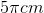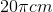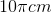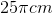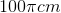Explanation:

The circumference of a circle is given by the equation:The radius is half the diameter, in this case half of 10cm is 5cm

Plug in 5cm for r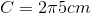Simplify to get the final answer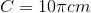### Example Question #4 : Diameter, Radius, And Circumference

If the diameter of a circle is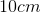, what is the area of the circle?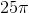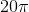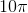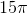Explanation:

Step 1: Recall the formula for an area of a circle...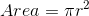.

Step 2: Given the diameter, find the radius..

We know that the diameter is twice the length of the radius...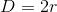Plug infor: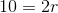Divide by 2: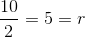Step 3: Now that we know the radius, plug the radius into the area formula..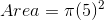Simplify: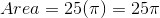### Example Question #5 : Diameter, Radius, And Circumference

Determine the circumference of the circle with an area of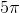.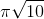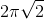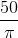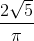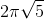Explanation:

Write the formula for the area of a circle.Substitute the area.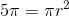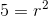Square root both sides to solve for radius.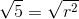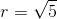Write the formula for circumference.The answer is: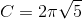### Example Question #6 : Diameter, Radius, And Circumference

Find the diameter of a circle if the circumference is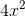.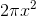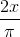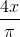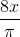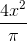Explanation:

Write the formula for the circumference of the circle.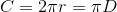Substitute the circumference into the equation.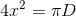Divide by pi on both sides to get the diameter.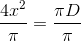The answer is:### Example Question #7 : Diameter, Radius, And Circumference

Find the area of a circle if the circumference is.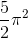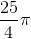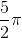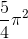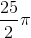Explanation:

Write the formula for the circumference of a circle.Substitute the circumference.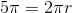Divide byto isolate the.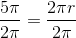The radius is: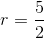Write the formula for the area of the circle.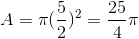The answer is: